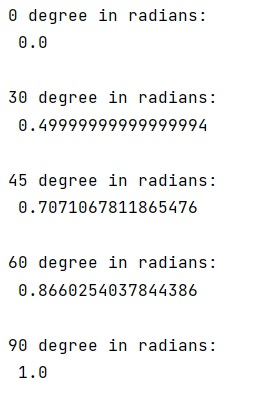# numpy.sin() function in degrees

Learn how to use numpy.sin() method and does it return the answer in degrees?
Submitted by Pranit Sharma, on February 10, 2023

NumPy is an abbreviated form of Numerical Python. It is used for different types of scientific operations in python. Numpy is a vast library in python which is used for almost every kind of scientific or mathematical operation. It is itself an array which is a collection of various methods and functions for processing the arrays.

## numpy.sin() function

Mathematically, sin is the ratio of the length of the opposite side to that of the hypotenuse in a right-angled triangle. The sine function is used to find the unknown angle or sides of a right triangle.
We can apply the sin function at different angles and it will give different values with each angle.

We need to understand the returned values of numpy.sin() function. We know that an angle can be measured in degrees or radians and since we already have our number (90) in degrees, we need to convert 90 from degree to radians or any other angle to radians and we need to do it before we calculate the sine for that angle.

Let us understand with the help of an example,

## Python code to demonstrate the example of numpy.sin() function in degrees

```# Import numpy
import numpy as np

# Calculate sine at different angles

```

Output: